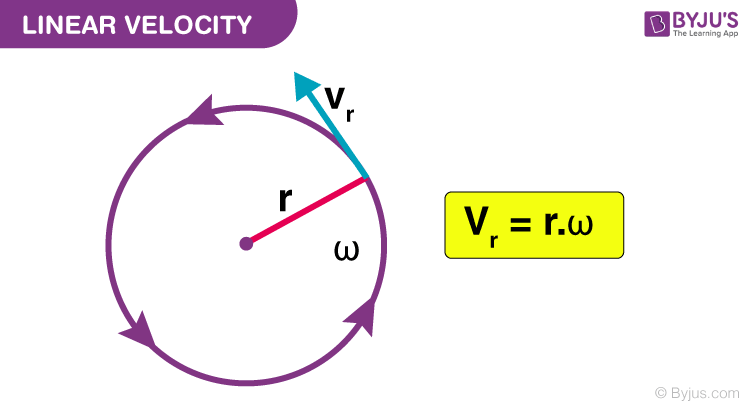# Linear Velocity

Velocity is the rate of change in an object’s position in a specific time range. When the object moves along a straight path, its velocity is termed linear velocity. It is given as the ratio of distance covered to time. It is denoted using V and measured using the S.I unit m/s. It is a vector quantity.

## What Is Linear Velocity?

Linear velocity is the measure of “the rate of change of displacement with respect to time when the object moves along a straight path.” It is a vector quantity. The dimension formula of linear velocity is [M]0[L]1[T]-1

Linear and angular velocities are related to the speed of an object based on the perspective chosen. Linear velocity is applied to an object that moves, whereas angular velocity applies to those that turn, such as a wheel, the earth’s revolution or a spinning top.

### Linear Velocity Formula

The linear velocity depends on the distance an object travels with respect to the time taken. The linear equation or the linear velocity formula is given by,

v = x/t

Where,

• v = Linear velocity
• x = distance covered
• t = Time taken to cover the distance(x).

### Linear Velocity units

Linear velocity is measured using SI unit meter per second or m/s

## Angular Velocity to Linear Velocity

Anything that moves or turns in the circular direction has both linear velocity and angular velocity. The angular velocity is the ratio of the angle traversed to the amount of time it takes to traverse that angle.Angular velocity gives an expression of angular displacement over time and can be expressed in radians or degrees. Angular velocity is determined with the above-mentioned equation.

To calculate the linear velocity from angular velocity, you can apply the formula, where –

r indicates the radius of the path taken.

### Constant Linear Velocity

When a body moves with constant speed without changing its direction, it is said to be moving with a constant linear velocity. Linear velocity indicates the direction is not changed, and constant shows that the magnitude remained constant.

Physics Related Topics:

## Frequently Asked Questions – FAQs

Q1

### What is linear velocity?

Linear velocity is defined as the vector quantity that denotes the rate of change of position of an object from its equilibrium position with respect to mass.
Q2

### How do you determine the magnitude of the linear velocity of point B on a link AB which is relative to point A?

Following is the equation that is used to determine the magnitude of the linear velocity of a point B on a link Ab relative to point A:
ω*AB
Q3

### What is a tangential component?

A tangential component is a component at which the centripetal component is parallel to the velocity of the particle.
Q4

### What is a radial component?

A radial component is a component at which the centripetal component is perpendicular to the particle’s velocity.
Q5

[ML2T-1]
Q6

### What does the rate of displacement mean?

The displacement rate means the body’s velocity, which is measured in metres per second.
Q7

### What is instantaneous velocity?

Instantaneous velocity is the velocity of the body at a given instant. The SI unit of instantaneous velocity is m.s-1.
Q8

### How do you determine the direction of the linear velocity of a body moving in a circle?

The direction of a linear velocity of a body moving in a circle is determined along the tangent.
Q9

### What is the angle between the linear velocity and the angular velocity of a body moving in a circle?

The angle between the linear velocity and the angular velocity of a body moving in a circle is 90°.
Q10

### Define linear velocity.

The rate of change of displacement with respect to the time when the object moves along a straight path.
Q11

### Write the expression for linear velocity.

The expression for linear velocity is
v=xt
Q12

### Write the dimensional formula of linear velocity.

The dimensional formula of linear velocity is [ML1T-1]
Q13

### State true or False. “Linear velocity is a scalar quantity.”

False. Linear velocity is a vector quantity.
Q14

### Write the type of motion involved in linear velocity.

Motion along a straight line.
Q15

### Write the SI unit of linear velocity.

SI Unit of linear velocity is m/s.
Q16

### Name the types of velocities involved in a circular motion.

Angular velocity and linear velocity.
Q17

### If an object covers 3 meters in 2 seconds along a straight. Calculate its linear velocity.

Substituting the values in v = x/t, we get linear velocity as 1.5 m/s.
Q18

424 m/h.
Q19

### An object is moving in a circular path. How will its linear velocity change if the diameter of the circular path is decreased by one-half?

Half.